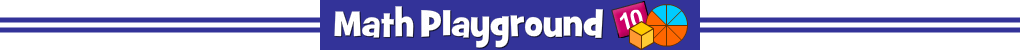Advertisement
Gap Zappers (Flash)
Gap Zappers - Learning Connections
Essential Skills
Problem Solving - complete mathematical puzzles

Common Core Connection for Grades 3 and 4
Recognize and generate simple equivalent fractions.
Explain why the fractions are equivalent by using a visual fraction model.
Decompose a fraction into a sum of fractions with the same denominator.
Compare two fractions with different numerators and different denominators.
Understand a multiple of a/b as a multiple of 1/b.
More Math Games to Play